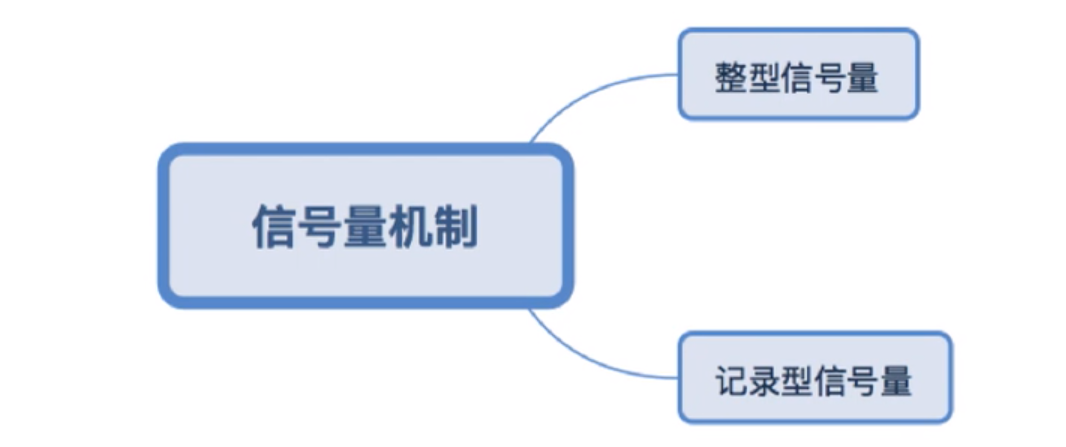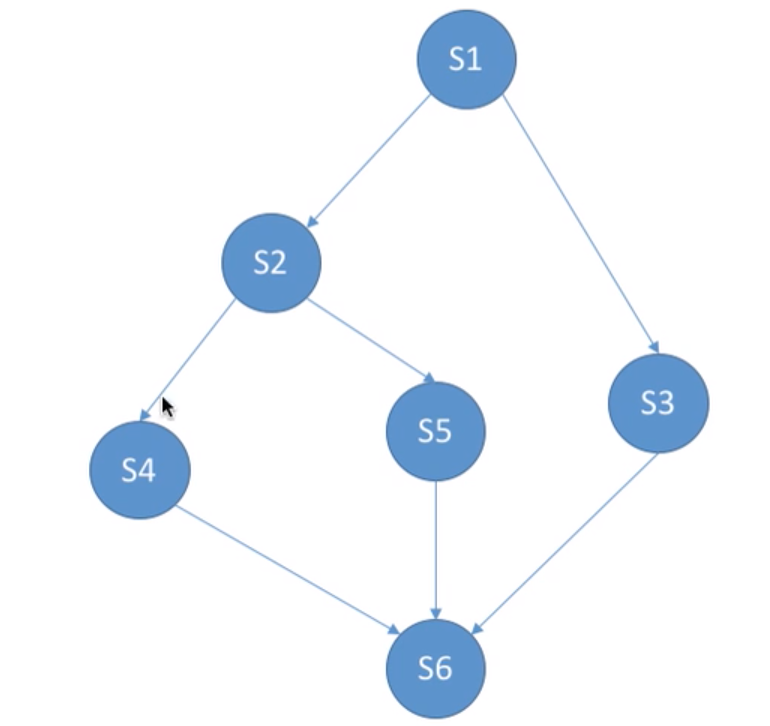# 操作系统自学（十二）信号量机制及用信号量机制实现进程互斥 同步 前驱关系

## 信号量机制### 整型信号量

int S=1;//初始化整型信号量S 表示当前系统中可用打印机资源数

void wait(int S){//wait原语 相当于进入区
while(S<=0);//如果资源数不够 就一直等待循环
S--;//如果资源数够 占用一个资源
}

void signal(int S){//signal 原语 相当于退出区
S++;//使用完资源之后 在退出区释放资源
}

……
wait(S);//进入区 申请资源

signal(S);//退出区 释放资源

wait(S);//进入区 申请资源

signal(S);//退出区 释放资源


### 记录型信号量

typedef struct{
int value;//剩余资源数
struct process *L;//等待队列
} semaphore;

//某进程想要使用资源的时候 通过wait原语申请
void wait (semaphore S){
S.value--;
if(S.value < 0){
block(S.L);
//如果剩余资源数不够，使用block原语使得进程从运行态转换到阻塞态
//并把挂到信号量S的等待队列中
}
}

//进程使用完资源之后，通过signal原语释放
void signal(semaphore S){
S.value++;
if(S.value<=0){
wakeup(S.L);
//释放资源之后，若还有别的进程等待这种资源，则使用wakeup原语唤醒等待队列中的一个进程
//该进程从阻塞态变为就绪态
}
}


## 信号量机制实现进程互斥 同步 前驱关系

### 进程互斥

//信号量机制实现互斥
semaphore mutex=1;//初始化信号量

p1(){
P(mutex);//使用临界资源之前需要加锁
临界区代码段
V(mutex);//使用临界区资源后需要解锁
}

p2(){
P(mutex);//使用临界资源之前需要加锁
临界区代码段
V(mutex);//使用临界区资源后需要解锁
}


P V必须成对出现

### 进程同步

semaphore S=0;//初始化同步信号量 初始值为0
P1(){
代码1;
代码2;
V(S);
代码3;
}

P2(){
P(S);
代码1;
代码2;
代码3;
}


### 前驱关系S1到S2对应a=0
S1到S3对应b=0
S2到S4对应c=0
S2到S5对应d=0
S4到S6对应e=0
S5到S6对应f=0
S3到S6对应g=0

p1(){
S1;
V(a);
V(b);
}
p2(){
P(a);
S2;
V(c);
V(d);
}
p3(){
P(b);
S3;
V(g);
}
p4(){
P(c);
S4;
V(e);
}
p5(){
P(d);
S5;
V(f);
}
p6(){
P(e);
P(f);
P(g);
S6;
}